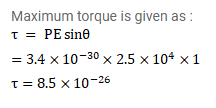# A sample of HCL gas is placed in an electric field ofQuestion:

A sample of HCL gas is placed in an electric field of $2.5^{\times 10^{4}} \mathrm{~N} / \mathrm{C}$. The dipole moment if each HCL molecule is $3.4^{\times 10^{-30}}$ C$\mathrm{m}$. Find the maximum torque that can act on a molecule.

Solution: